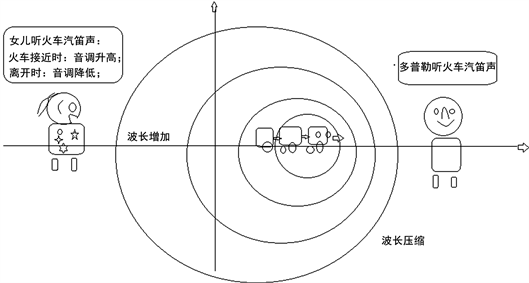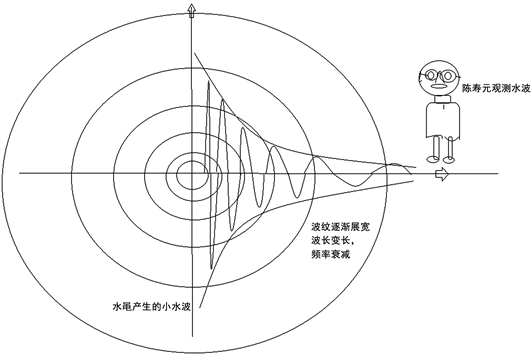# 陈寿元效应与多普勒效应在天文学上对决The Shouyuan Chen Effect and Doppler Effect Confronted in Astronomy

DOI: 10.12677/AAS.2019.73006, PDF, HTML, XML, 下载: 9,577  浏览: 20,737

Abstract: This paper first introduces the concepts of Doppler Effect and Chen Shouyuan Effect. Then, the ex-planation of astronomical information by the two effects is discussed. Through comparison, the re-sults of the two effect treatments are comparable. The Doppler Effect treats the redshift of the un-iverse as a big bang, an explosion at a point where the universe originated. The conclusion is more absurd than Ptolemy's "the universe revolves around Earth". The Chen Shouyuan effect treats the redshift of the universe as the energy loss of light wave during the long-distance propagation of light wave in space, resulting in extremely weak attenuation of the frequency of light wave energy factor with the increase of the propagation distance, thus forming the so-called redshift. Redshift essentially means that the spectral frequency moves towards the red end, that is, the frequency de-creases. And decay according to exponential law, first-order approximation, is a linear relationship, consistent with Hubble’s observation law, but the name of the coefficient is different. The possibility of redshift of universe caused by Chen Shouyuan effect is much greater than Doppler Effect. Its arguments are more in line with modern science, especially communication science, electromag-netism and physics. Everyone is concerned about "if the Chen Shouyuan effect exists, why has it not been measured before". Because the effect is too weak, the precision of previous experiments is not enough. The weak attenuation of the frequencies of water waves, sound waves and electromagnetic waves is measured by adopting a new method of multi-experiment design. The conclusion will lead to scientific criticism and negation of the big bang theory.

1. 引言

1929年，哈勃测量到遥远星系发来的光，其谱线有向红的一端移动的现象(实际上，就是光频率随传播距离而衰减的结果)。但是，由于受传统科学的影响，错过频率衰减的认定最佳时期，而采用1846年多普勒发现的效应给出解释，并推理出宇宙膨胀、宇宙大爆炸等怪论。1964年，彭齐亚斯和威尔逊在巨型号角天线上，测量到2.7的微波背景辐射，被定义为是宇宙大爆炸的余辉；1989年11月，约翰·马瑟、乔治·斯穆特主持升空COBE卫星，测量到符合温度为2.726 K的黑体辐射谱的背景辐射，再一次认定为大爆炸余辉。1999年，索尔·波尔穆特、布莱恩·施密特和亚当·里斯研究超新星，发现亮度衰减更快、红移更大，推理出宇宙加速膨胀  -  的论调，使得全人类处于迷茫、争论、质疑之中。在目前测量技术实验手段下，机械波、电磁波以及声波、光波在传播过程中，确实没有测量到频率衰减。但是其频率也是波能量因子，不衰减，理论上讲不通，极有可能测量精度没有达到频率衰减的测量精度要求。陈寿元效应  -  ：频率与振幅一样，都是波能量因子。在其传播过程中，波能量损失，既可以导致振幅衰减，也可以导致频率衰减。并通过精密实验测量到频率衰减。

2. 多普勒效应

1842年一天，多普勒带女儿在火车道口边玩耍，注意到火车声音变化，火车由远到近开来时，汽笛发出的声音，听到声音音调升高，再由近到远离开时，汽笛声音音调降低的现象，如图1所示。Figure 1. Changes of wavelength or frequency in the wave source moving observed by doppler

$Z=\frac{{\lambda }_{O}-{\lambda }_{S}}{{\lambda }_{S}}=±\frac{V}{C}$ (2.1)

1845年，荷兰气象学家拜斯·巴洛特在让一队喇叭手，站在一辆从荷兰乌德勒支附近疾驶而过的敞篷火车上吹奏，他让音乐家在站台上，用耳朵辨别、测到了音调的改变。这是科学史上最有趣的实验之一。

1868年，英国天文学家威廉·哈金斯首次测出了恒星相对于地球的运动速度。在1871年，利用太阳的自转测出在可见光太阳光谱的夫朗和斐谱线在红光有0.1 Å的位移。在1901年，Aristarkh Belopolsky在实验室中利用转动的镜片证明了可见光的红移。在1912年开始的观测，Vesto Slipher发现绝大多数的螺旋星云都有不可忽视的红移。

1929年，埃德温·哈勃发现这些星云(现在知道是星系)的红移和距离有关联性，也就是哈勃定律。 这些观察在今天被认为是造成宇宙膨胀大爆炸理论的强而有力证据。

3. 陈寿元效应

3.1. 定义

3.2. 陈寿元效应发现过程

3.3. 陈寿元效应的理论论述

${\varnothing }_{B}-{\varnothing }_{A}=-E\left(B-A\right)$ (3.1)

(3.1)式中：E——能场强度，克服能量耗散而做功。波动从A点传递到B点的必要条件： ${\varnothing }_{A}>{\varnothing }_{B}.$

A点的能场强度 ${E}_{A}$

${E}_{A}=-{\mathrm{lim}}_{B\to A}\left(\frac{{\varnothing }_{B}-{\varnothing }_{A}}{B-A}\right)$

E——能场强度：可用能位函数的负梯度来表示：

$E=-\nabla \varnothing$ (3.2)

$Y\left(x,t\right)=A\left(x\right)\mathrm{cos}\left(\omega \left(x\right)t-kx\right)$ (3.3)

(2.3)式中：A(x)——波的振幅，通常随传播距离而衰减，是X的函数。ω(x)角速度，目前认为它不随传播距离变化，是不变量。但是前面的分析，振源能量与频率平方成正比。能量是要消耗，扩散、色散。能量在空间上的散开，表现占用更大面积或更大的体积空间，使波长变长。在时域上，能量散开意味着占用更多的时间段,使振动的周期有延长的趋势。

${\upsilon }_{y}=\frac{\partial Y\left(x,t\right)}{\partial t}=-A\left(X\right)\omega \left(X\right)\mathrm{sin}\left(\omega t-kx\right)$ (3.4)

${\text{cp}}_{y}\left(x,t\right)=\frac{1}{2}{\upsilon }_{y}^{2}=\frac{1}{2}{A}^{2}\left(x\right){\omega }^{2}\left(x\right){\left(\mathrm{sin}\left(\omega t-kx\right)\right)}^{2}$ (3.5)

(3.5)式表明波动的能位函数与波动的频率平方成正比。

$\text{cp}\left(x\right)=\frac{1}{4}{A}^{2}\left(x\right){\omega }^{2}\left(x\right)$ (3.6)

$\frac{\text{d}p}{\text{d}x}=-\alpha p$

$p\left(x\right)=p\left(0\right){\text{e}}^{-\alpha x}$ (3.7)

(3.6)式代入(3.7)式，得

$A\left(x\right)\omega \left(x\right)=A\left(0\right)\omega \left(0\right){\text{e}}^{-\frac{1}{2}\alpha x}$ (3.8)

1) 若信号传输过程中，频率不变，即：

$\omega \left(x\right)=\omega \left(0\right)$

2) 若信号传输过程中，振幅保持不变，即：

$A\left(x\right)=A\left(0\right)$

3) A(x)，ω(x)共同分担信号的衰减量：

$A\left(x\right)=A\left(0\right){\text{e}}^{-\frac{1}{4}\alpha x}$

$\omega \left(x\right)=\omega \left(0\right){\text{e}}^{-\frac{1}{4}\alpha x}$ (3.11)

4) $A\left(x\right) (3.12)

5) $\omega \left(x\right)<\omega \left(0\right){\text{e}}^{-\frac{1}{2}\alpha x}$ (3.13)

6) 更一般现实情况，A(x)衰减快，ω(x)衰减慢。波的形态分三段：波能量密度很大，随传播距离，振幅衰减为主，频率衰减为辅——称为波状态；波能量密度小，振幅衰减为辅，频率衰减为主——量子状态；波能量密度非常小，振幅、频率都快速衰减为零——波消亡态。从 $k{\text{e}}^{-\frac{1}{2}\alpha x}$${\text{e}}^{-\frac{1}{4}\alpha x}$ 之间变化。

7) 衰减系数的关系

$\phi \left(x\right)=\phi \left(0\right){\text{e}}^{-2\left(\alpha +\beta \right)x}$

$A\left(x\right)=A\left(0\right){\text{e}}^{-\alpha x}$

$f\left(x\right)=f\left(0\right){\text{e}}^{-\beta x}$

3.4. 陈寿元效应的频率随传播距离、衰减示意图Figure 2. Shouyuan Chen effect: wavelength increases with propagation distance

4. 陈寿元效应与多普勒效应在天文学上应用对决

4.1. 宇宙红移的解释

$Z=\frac{{\lambda }_{O}-{\lambda }_{S}}{{\lambda }_{S}}=HD$

$V=C\ast \frac{{\lambda }_{O}-{\lambda }_{S}}{{\lambda }_{S}}=CHD={H}_{0}D$

${\lambda }_{O}={\lambda }_{S}{\text{e}}^{\alpha d}$

$Z=\frac{{\lambda }_{O}-{\lambda }_{S}}{{\lambda }_{S}}={\text{e}}^{\alpha d}-1\approx \alpha d$

4.2. 超新星观测结果的解释

1999年，索尔·波尔穆特、布莱恩·施密特和亚当·里斯研究超新星，发现亮度衰减更快、红移更大的观测结果。

4.3. 对太阳光的解释

$\begin{array}{c}\Delta f=Zd=f\alpha d\\ =1.5×{10}^{11}×\left(0.8\text{\hspace{0.17em}}\text{-}\text{\hspace{0.17em}}5\right)×{10}^{-26}×\left(3.9\text{\hspace{0.17em}}\text{-}\text{\hspace{0.17em}}8.6\right)×{10}^{14}\\ =0.5\text{\hspace{0.17em}}\text{-}\text{\hspace{0.17em}}3\end{array}$

4.4. 最近恒星光的解释

$\Delta f=f\alpha d=4\ast c\ast t\alpha f=1.89×{10}^{5}\text{\hspace{0.17em}}\text{Hz}$

4.5. 银河系星光变化的解释

4.6. 河外星系光频率变化的解释

$d=\frac{Z}{H}=\frac{\Delta f}{f\alpha }$

4.7. 背景辐射

5. 结束语

  李宗伟, 肖兴华. 天体物理学[M]. 北京: 高等教育出版社, 2000: 426-472.  陈寿元. 信息物理[M]. 济南: 山东地图出版社, 2008.  凯德洛夫, 等, 主编. 物理学的方法论原理[M]. 柳树滋, 等, 译. 北京: 知识出版社, 1990: 447.  Hewitt, P.G. (1989) Conceptual Physics. The Champagne Company, Birmingham.  程守洙, 江之水, 编. 普通物理学[M]. 北京: 高等教育出版社, 1961.  Guru, B.S. (2005) Electromagnetic Field Theory Fundamentals. 2nd Edition, China Machine Press, Beijing.  漆安慎, 等. 普通物理学教程: 力学[M]. 第2版. 北京: 高等教育出版社, 2005.  郭奕玲, 等. 物理学史[M]. 北京: 清华大学出版社, 1993.  曾心传, 等. 震动理论基础[M]. 北京: 地震出版社, 2013.  管平, 等. 数学物理方法[M]. 北京: 高等教育出版社, 2001.  蔡圣善, 等. 电动力学[M]. 第2版. 北京: 高等教育出版社, 2002.  贾起民, 等. 电磁学[M]. 第2版. 北京: 高等教育出版社, 2001.  刘增基, 等. 电磁场与电磁波[M]. 西安: 电子科技大学出版社, 2002.  Hiziroglu, H.R. (2004) Electromagnetic Field Theory Fundamentals. China Machine Press, Beijing.  柯亨玉. 电磁场理论[M]. 北京: 人民邮电出版社, 2004.  范寿康, 等. 微波技术与微波电路[M]. 北京: 机械工业出版社, 2003.  Communications Week, 1993, 9.  Rappaport, T.S. (1996) Wireless Communications Principles & Practice. Prentice Hall, Upper Saddle River, NJ.  郭梯云, 邬国杨, 李建东. 移动通信[M]. 西安: 西安电子科技大学出版社, 2001.  李兆玉, 何维, 戴翠琴. 移动通信[M]. 北京: 电子工业出版社, 2017.  陈显治, 等. 现代通信技术[M]. 北京: 电子工业出版社, 2004.  赵洪波, 卜益民, 陈风娟. 现代通信技术概论[M]. 北京: 北京邮电大学出版社, 2003.  张传福, 彭灿, 胡熬, 刘晓甲, 卢辉斌. CDAM移动通信网络规划设计与优化[M]. 北京: 人民邮电出版社, 2006.  苏华鸿, 孙儒石, 等. 蜂窝移动通信射频工程[M]. 北京: 人民邮电出版社, 2005.  冯玉珉. 通信系统原理[M]. 北京: 清华大学出版社, 2003.  Saml Tabbans. 无线移动通信网络[M]. 李新付, 等, 译. 北京: 电子工业出版社, 2001.  孙宇彤, 等. CDMA空中接口技术[M]. 北京: 人民邮电出版社, 2004.  Rhee, M.Y. (2002) CDMA Cellular Mobile Communications and Network Security. Prentice Hall, Upper Saddle River, NJ.  Feher, K. (1995) Wireless Digital Communication. Prentice Hall, Upper Saddle River, NJ.  GSM Recommendation 03.01, ETS300 521-1994. 1994, 1.  牛顿. 自然哲学之数学原理[M]. 王克迪, 译. 北京: 北京大学出版社, 2006.  [美] Seeds, M.A. (1998) Astronomy. The Solar System and Beyond. Wadsworth Publishing Company, Belmont, CA.  庄德新, 聂清香. 天文学[M]. 济南: 山东大学出版社, 2002.  伽利略. 关于托勒密和哥白尼两大世界体系的对话[M]. 周熙良, 等, 译. 北京: 北京大学出版社, 2006.  哥白尼. 天体运行论[M]. 叶式辉, 译. 北京: 北京大学出版社, 2006.  李宗伟, 肖兴华. 天体物理学[M]. 北京: 高等教育出版社, 2000.  陈宇, 陈寿元. 信道天文学(简介): 现代通信模式处理天文信号[J]. 天文与天体物理, 2019, 4(1): 25-34.  Dampier, W.C. A History Science. 科学史及其哲学与宗教的关系[M]. 李珩, 译. 桂林: 广西师范大学出版社, 2001: 105-110.  杜功焕, 等. 声学基础[M]. 第2版. 南京: 南京大学出版社, 2001.  曽心传, 等. 震动理论基础[M]. 北京: 地震出版社, 2013.  易克初, 等. 语音信号处理[M]. 北京: 国防工业出版社, 2000.  陈熙谋. 光学. 近代物理学[M]. 北京: 北京大学出版社, 2002.  刘增基, 等. 光纤通信[M]. 西安: 电子科技大学出版社, 2001.  易搏藤, 等. 电磁学简明教程[M]. 北京: 北京师范大学出版社, 2001.  约翰∙克劳斯. 天线[M]. 章文勋, 译. 北京: 电子工业出版社, 2004.  Yüksel, H., Kistler, M.D., Beacom, J.F. and Hopkins, A.M. (2008) Revealing the High Red-Shift Star Formation Rate with Gamma-Ray Bursts. The Astrophysical Journal, 683, L5. https://doi.org/10.1086/591449  Wang, F.Y. and Dai, Z.G. (2009) High Red-Shift Star Formation Rate Up to Z~8.3 Derived from Gamma-Ray Bursts and Influence of Background Cosmology. Monthly Notices of Royal Astronomical Society, 400, L10-L14. https://doi.org/10.1111/j.1745-3933.2009.00751.x  Dado, S. and Dar, A. (2013) Long Gamma Ray Bursts Trace the Star Formation History. Astrophysics, Arxiv: 1307.5556.  Wang, F.Y. (2013) The High Red-Shift Star Formation Rate Derived from Garmma-Ray Bursts Origin and Cosmic Reionization. Astronomy & Astrophysics, 556, Article No. A90. https://doi.org/10.1051/0004-6361/201321623  Hopkins, A.M. and Bencom, J.F. (2006) On the Normalization of the Cosmic Star Formation History. The Astrophysical Journal, 651, 142-154. https://doi.org/10.1086/506610  Sokolov, V.V. (2013) The Gamma-Ray Bursts and Core-Collapse Superno-vovae-Global Star Forming Rate Peaks at Large Red-Shifts. Astrophysics, Arxiv: 1310.7730.  Perlmutter, S., et al. (1999) Measurements of Ω and Λ from 42 High-Redshift Supernovae. The Astrophysical Journal, 517, 565-586.  陈寿元. 万有阻力定律的几点天文观测验证[J]. 山东师范大学学报(自然科学版), 2000(4): 16.  陈寿元. 相对静止论[J]. 山东大学学报(工学版), 2002, 32(4): 396-400.  陈宇, 陈寿元. 基于现代通信模型的遥远星系光色变化的研究[J]. 山东师范大学学报(自然科学版), 2019, 34(1): 61-69.  陈宇, 陈寿元. 天文信息的处理模式研究[J]. 天线学报, 2018, 7(4): 25-34.  陈寿元. 频率衰减: 哈勃红移——陈寿元效应[J]. 科技信息, 2017(11): 50.  陈宇, 陈寿元. 信道天文学(简介): 通信模式处理天文信号[J]. 天文宇天体物理, 2019(1).  陈寿元. 基于陈寿元效应的水波频率衰减、红移实验装置及方法[P]. 中国专利.  陈寿元. 基于陈寿元效应的声波频率衰减、红移实验装置及方法[P]. 中国专利.  陈寿元. 基于陈寿元效应的电磁波频率衰减、红移实验装置及方法[P]. 中国专利.  陈寿元. 基于陈寿元效应的微波频率衰减、红移实验装置及方法[P]. 中国专利.  陈寿元, 陈宇. 一种基于陈寿元效应的电磁波红移测量系统及方法[P]. 中国专利.  陈寿元, 陈宇. 一种基于陈寿元效应的声波红移测量系统及方法[P]. 中国专利.  陈寿元, 陈宇. 一种基于陈寿元效应的水波红移测量系统及方法[P]. 中国专利.  陈寿元, 孙跃鲁, 李文健. 远距离通信系统与方法[P]. 中国专利.  陈寿元, 陈宇. 一种基于光波红移的天文观测望远镜[P]. 中国专利.  陈寿元. 频率衰减——陈寿元效应[Z]. https://v.youku.com/v_show/id_XMzQ5NDkxMTcyOA==.htm  陈寿元. 信道天文学[Z]. https://v.youku.com/v_show/id_XNDA1MDg1ODIxNg==.html?spm=a2h0j.11185381.listitem_page1.5~A  陈寿元. 声波红移实验原理[Z]. https://v.youku.com/v_show/id_XMzY0MDU0NDc2OA==.html?spm=a2h0j.11185381.listitem_page1.5!3~A  陈寿元. 声波波长随传播距离而缓慢增加的实验[Z]. https://v.youku.com/v_show/id_XMzY0MDQ3NDExNg==.html?spm=a2h0j.11185381.listitem_page1.5!4~A  陈寿元. 宇宙大爆炸质疑[Z]. https://v.youku.com/v_show/id_XMzYwOTA4ODA3Mg==.html?spm=a2h0j.11185381.listitem_page1.5!8~A  陈寿元. 当代大学生对陈寿元效应视频观后感[Z]. https://v.youku.com/v_show/id_XMzU4MjUzNDU2NA==.html?spm=a2h0j.11185381.listitem_page1.5!19~A  陈寿元. 哈勃星系红移新解释[Z]. https://v.youku.com/v_show/id_XMzU3MDQ3NDUzMg==.html?spm=a2h0j.11185381.listitem_page1.5!24~A  陈寿元. 陈寿元效应[Z]. https://v.youku.com/v_show/id_XMzU0ODcwMjM2MA==.html?spm=a2h0j.11185381.listitem_page1.5!28~A  陈寿元. 宇宙大爆炸与水面的故事[Z]. https://v.youku.com/v_show/id_XMzU0NzcxOTE2OA==.html?spm=a2h0j.11185381.listitem_page1.5!29~A  陈寿元. 星光之谜[Z]. https://v.youku.com/v_show/id_XMzY0Njk4ODAyOA==.html?spm=a2h0j.11185381.listitem_page1.5  陈宇, 陈寿元. 基于陈寿元效应对宇宙大爆炸论的批判[J]. 天文与天体物理, 2019, 7(3): 40-52.  戴文赛. 太阳系演化学[M]. 北京: 科学出版社, 1980.  李艳平, 申先甲. 物理学史教程[M]. 北京: 科学出版社, 2003: 102.  凯德洛夫, 等, 主编. 物理学的方法论原理[M]. 柳树滋, 等, 译. 北京: 知识出版社, 1990.  关士续, 等. 自然辩证法概论[M]. 北京: 高等教育出版社, 2002: 49-52.